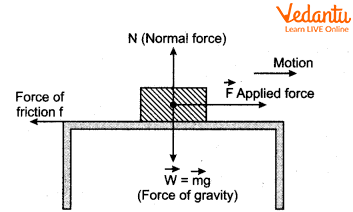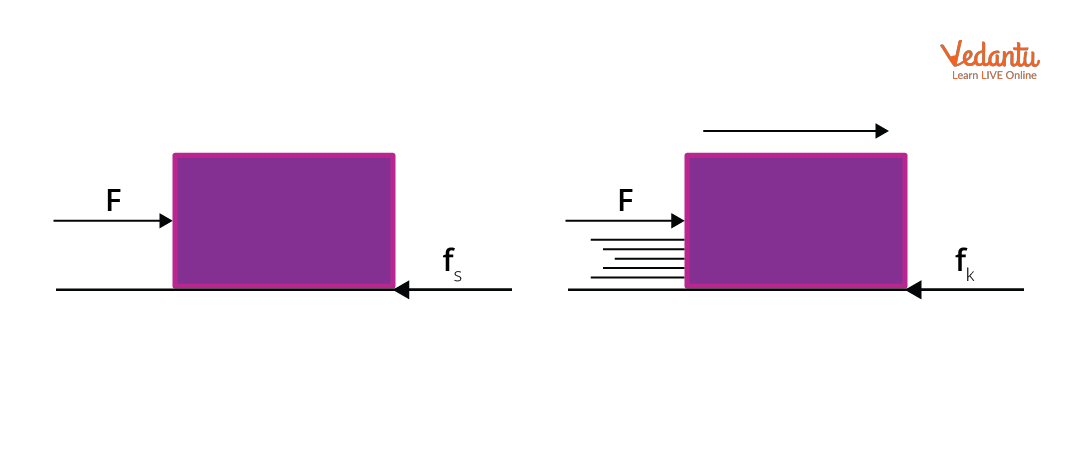Courses
Courses for Kids
Free study material
Free LIVE classes
More

# Friction, Types, and Laws Related to It - JEE Important TopicLIVE
Join Vedantu’s FREE Mastercalss

## Friction: Resisting Force

In our daily lives, we come across so many situations where two surfaces move past each other. When an object slides or rolls past another object, a force needs to be applied to overcome the resistance. Friction is the resisting force that prevents the sliding or rolling of one object over the other. Even though friction is a resisting force, it is required for walking without slipping, holding objects, working of brakes, and many other purposes. Friction is the resisting force which acts parallel to the surface of contact, and it resists the relative motion of an object. The main cause of friction is surface roughness.

## Definition of Friction

Friction is a contact force that opposes the relative motion of any two surfaces. Both of the bodies experience a contact force that opposes the relative motion of the surfaces when the surface of one body glides across the surface of the other body. The frictional force is this opposing force. The intermolecular interaction that results from the elastic characteristics of the matter is what causes friction. At the intersection of the surfaces of two bodies, the frictional force acts tangentially.

The nature of the two surfaces in contact, the normal force acting on those surfaces, and the actual area of contact between the surfaces, all affect friction.

## What Does Friction Produce?

Friction between two surfaces produces heat. When we rub our palms together, after some time, they feel warm. Also, a mixer jar after operating, sometimes gets heated. The surfaces of moving objects or machine parts produce heat that causes wastage of energy.  Friction also causes the wear and tear of surfaces which are moving past each other. For example, we can see the wearing of moving parts such as screws, ball bearings, soles of shoes, and so on.

## Laws of Friction and Coefficients of Friction

Consider an object which is initially at rest. The forces acting on the object are shown in the diagram. When we try to move the object, initially, it doesn't move. This is due to the friction between the object and the surface that it is kept on. The frictional force depends on the normal force between the object and the surface.Free Body Diagram of the Object

Mathematically, we can express the frictional force by using the law of limiting friction.

When the applied force exceeds a definite limit, the body begins to move. Experimentally, it is found that the limiting value of static friction fs is independent of the area of contact, and it varies proportionally with the normal force N. This means that:

\begin{align} &f_{s} \propto N \\ &f_{s}=\mu_{s} N \end{align}

The constant of proportionality μs introduced in the above expression is known as the coefficient of static friction. In simpler terms, static friction is the friction that acts between two bodies that are not slipping over each other.

Now, when the applied force is greater than the static friction, the object begins to slip.

Frictional force that resists the relative motion between surfaces in contact is called kinetic friction. The mathematical term for kinetic friction can similarly be written as:

\begin{align} &f_{k} \propto N \\ &f_{k}=\mu_{k} N \end{align}

The constant of proportionality μk introduced is the coefficient of kinetic friction.Static and Kinetic Friction Force Acting on an Object

The values of the coefficients of friction are calculated experimentally. It has been experimentally verified that

$\mu_{s}>\mu_{k}$

The coefficient of friction indicates the amount of interaction between the two surfaces. It is the ratio of the frictional force resisting the motion to the normal force acting on surfaces. A higher value of coefficient of friction means that more friction force is present relative to the normal force and more will be the resistance for relative motion. The coefficient of friction depends on the nature of the surfaces that are in contact, i.e. if the surfaces are rough, polished, dry or wet. It also depends on the materials that are in contact.

## Types of Friction

Earlier, we discussed what friction is. Now, let us take a look and understand the types of friction. Friction is classified into four types:

1. Static Friction: It is the type of friction which acts when two bodies are at rest. Static friction helps to keep the objects in the state of rest. For example: object at state of rest.

2. Dynamic or Kinetic Friction: When two surfaces of objects are moving, the friction caused is called dynamic friction. It is slightly smaller than the static friction. The two types of dynamic friction are:

1. Sliding Friction: When two surfaces slide, the friction generated is sliding friction. For example: sliding friction operates between objects which slide on a slanting surface.

2. Rolling Friction: When one body rolls over the surface of another body, the resistance to its motion is called rolling friction, which is less than the sliding friction as rolling reduces friction. For example: when the ball rolls down the hill.

1. Fluid Friction: When two layers of fluid move opposite to each other, the fluid friction arises. It is called viscosity. The frictional force on an object in a fluid is called drag. For example: water pushing against the swimmer's body as he swims in water.

## Factors That Affect Friction

There are different factors of friction that affect the friction generated when objects move. Let us see which factors influence the frictional force.

1. Smoothness of the Surfaces in Contact: If the surface of the objects in contact is smooth, then the friction will be less, and they can move past easily. However, if the surfaces are rough, then the friction will be more and the object will resist the motion.

2. Weight of Object: To move heavier objects, greater force is required as compared to lighter objects. This is because the friction offered by heavy objects is more.

3. Nature of Fluid and the Shape of the Object: When an object is in any fluid, the frictional force on the object will depend on the nature of fluid and shape of the object. The birds and fish have streamlined body shapes to overcome the fluid friction. The same concept is used to design aeroplanes, racing cars, etc.

## Applications of Friction

1. Friction helps to walk. Without friction, we also can’t stand stationary.

2. Friction helps to write on paper or on blackboards using a pen or chalk.

3. We can fix a nail into the wall because of friction between surfaces.

4. The brake system in vehicles is made on the basis of the concept of friction.

5. The pulley system works with the help of friction.

6. Friction helps to make fire by rubbing stone or rubbing match stick to match box.

## Ways to Reduce Friction

1. Polishing the rough surface.

2. Lubricating the surfaces of moving parts in engines, mechanical devices etc. by a proper lubricant.

3. Coating the metal surfaces in contact with soft material.

4. Convert sliding friction to rolling friction as in the case of wheels attached to trolleys.

## Conclusion

Frictional force opposes the relative motion between two surfaces which are in contact. It is a tangential force. The two main types of friction are static friction; which acts between surfaces at rest and kinetic friction; which acts when surfaces slide with each other. There are three types of kinetic friction: sliding friction, rolling friction and fluid friction. Friction is affected by the smoothness of the surfaces in contact, weight of the object kept over the surface and also the shape of the object and the nature of fluids in case of fluid friction. Friction can be reduced by application of lubricants, polishing rough surfaces, coating metal parts with soft material etc.

Last updated date: 17th Sep 2023
Total views: 128.1k
Views today: 3.28k

## FAQs on Friction, Types, and Laws Related to It - JEE Important Topic

1. Define limiting friction.

Limiting friction is the maximum force of static friction which is attained by a body that just started moving over another body’s surface, when in contact with each other. It is the friction acting between two static surfaces. It acts tangential to the two surfaces that are in contact. The limiting friction is independent of the shape and area of the surfaces, but depends on  material, nature of the surfaces in contact and smoothness. When force applied exceeds the limiting friction, the body starts to move. Limiting friction is the product of coefficient of friction to normal force.

2. What are the disadvantages of friction?

Friction in moving parts is the major cause for energy wastage. Friction in the moving part converts a part of kinetic energy to heat. To overcome the frictional resistance between the components of any machine, an extra amount of power is wasted.

Also, friction between moving surfaces causes wear and tear of moving parts, engines, turbines and so on. Friction also causes reduction in speed,  sparks, overheating and machine breakdown. The workers have to use more energy while doing work because of friction means extra effort is required to do any work.